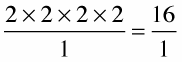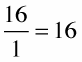##### Algebra II For Dummies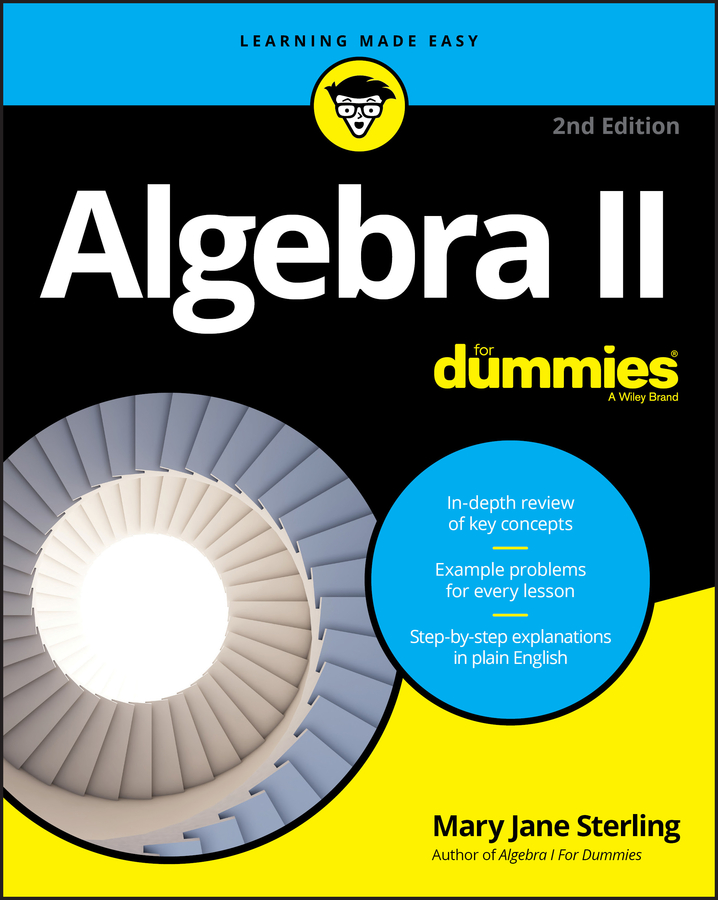Knowing how to multiply fractions will help when it comes to multiplying two mixed numbers. This is because when multiplying mixed numbers, you first have to change them to improper fractions. You then multiply the numerators and denominators, and reduce the results, if possible. Then, at the end, you can change the fraction back to a mixed number.

Example: Sadie worked 10 2/3 hours at time-and-a-half. How many hours will she get paid for?

1. Change all the mixed numbers to improper fractions.

In Sadie’s case, you have two mixed numbers, 10 2/3 and 1 1/2, that need to become improper fractions. Follow this formula to convert each mixed number into an improper fraction: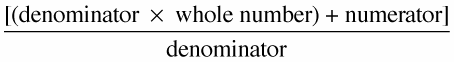In a fraction, the Numerator is always North of (above) the fraction line. The Denominator is always Down below the fraction line.

You convert the mixed numbers to improper fractions as follows: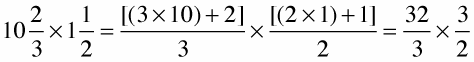2. Reduce the fractions.

To do this, break down both the numerator and denominator of each fraction into their prime factors (shown below in parentheses):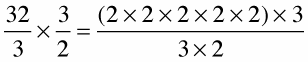3. Cross out any common factors.

In this example, you cross out 2 and 3 because they’re common factors — that is, they appear in both the numerator and denominator: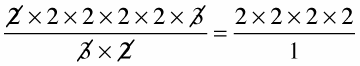4. Multiply the numerators together and the denominators together.